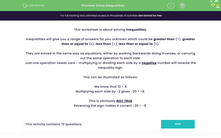# Solve Inequalities

In this worksheet, students will practise solving inequalities.Key stage:  KS 3

Curriculum topic:   Algebra

Curriculum subtopic:   Understand Expressions, Equations, Inequalities, Terms and Factors

Difficulty level:#### Worksheet Overview

This activity is about solving inequalities.

Inequalities will give you a range of answers for an unknown which could be greater than (>), greater than or equal to (≥), less than (<), less than or equal to (≤).

They are solved in the same way as equations, either by working backwards doing inverses, or carrying out the same operation to each side.

For example:

4x - 6 > 26

Solve it exactly as if it were an equals sign.

4x - 6 + 6 > 26 + 6

4x > 32

Divide both sides by 4:

x > 8

So, we know that x > 8

Just one operation needs care - multiplying or dividing each side by a negative number will reverse the inequality sign.

This can be illustrated as follows:

We know that 10 > 4

Multiplying each side by -2 gives -20 > -8

This is obviously NOT TRUE

Reversing the sign makes it correct -20 < -8

Let's have a go at some questions now.

### What is EdPlace?

We're your National Curriculum aligned online education content provider helping each child succeed in English, maths and science from year 1 to GCSE. With an EdPlace account you’ll be able to track and measure progress, helping each child achieve their best. We build confidence and attainment by personalising each child’s learning at a level that suits them.

Get started••••